MBCAA Observatory

# VX Hya time keeping

## Observations: 10, 11, 13, 16, 17, 18 March 2005

### Abstract

Time-series for this pulsating star with multiple oscillations are obtained. They are compared with the Fitch (1966) ephemeris: the matching is still good but with a delay of 1.4 hour.

### Introduction

VX Hydrae is a pulsating star of the delta Scuti type. It shows multiple periodicities.

### Observations

Time-series were carried out with a 203 mm SC telescope, Johnson V and B filters used alternatively and a SBIG ST7E camera (KAF401E CCD). 248 images were obtained with the V filter, 241 with the B one. Each exposure has a duration of 200 seconds.

The comparison star is Tycho 5482-01347. However, Tycho2 does not give reliable B magnitudes for this field. The adopted magnitudes for the comparison are then B=12.172 V=11.580.

The check star is GSC 5482-01054 with the observed magnitudes V=12.852 (average 1-sigma statistical uncertainty 0.019, standard deviation 0.024) and B=13.432 (0.039, 0.046).

The light curves show patterns that are very different from one session to another. Examples: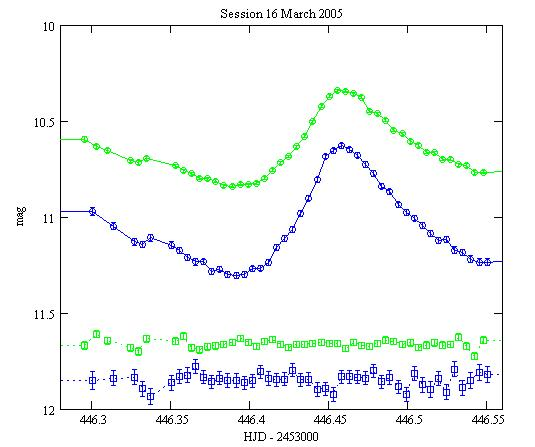Green: with the V filter, Blue: with the B one.
Circles: VX Hya magnitudes, Squares: check star magnitudes shifted by -1.2 mag in V, -1.6 in B.
The error bars are the +/- 1-sigma statistical uncertainties.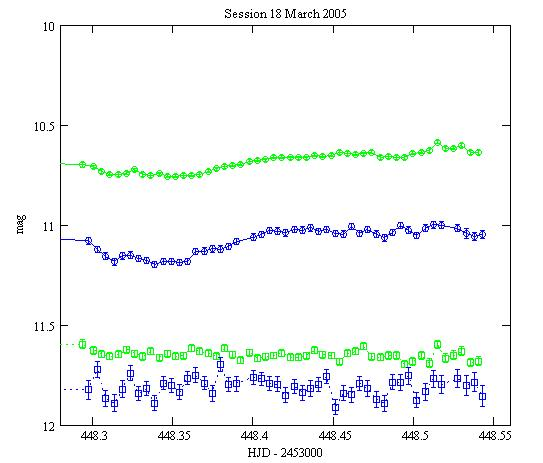All the light curves are here.

### Checking the ephemeris

VX Hya was monitored from 1954 to 1965 and a model of the light curve was derived (Fitch 1966). This model involves 2 oscillations:

n1=4.476495 1/day (period=5.36 h)
n2=5.789730 1/day (period=4.15 h)
T=2,435,000.0 (14 Sept 1954)

and a long-period modulation:

frequency nL=0.000227 1/day (period=12 yr)
TL=2,434,860.0 (27 Apr 1954)

the following way:with <V(t)>=10.696 mag the average magnitude. The coefficients a,b,c,d for the 1955-1959 period are given below. They do not fit correctly the 1964-1965 period.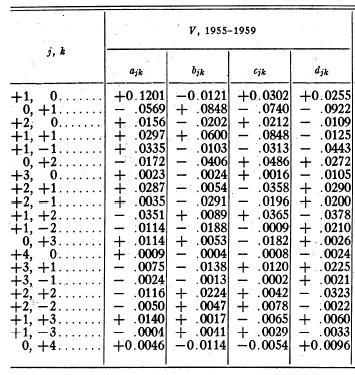Although the above model is good only for the 1955-1959 period, I tried to use it with my observations. It appears that the phase and the amplitude are not right. I fitted T by hand and computed <V(t)> and obtained a modelled light curve pretty close to the observations:

T = 2,435,000.0595 that is 1.428 hour late
<V(t)> = 10.634.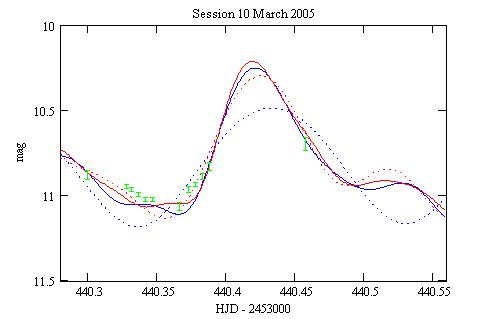Blue dot: the first order term only; Red dot: 1st+2nd terms; Blue: 1+2+3; Red: all 4 terms.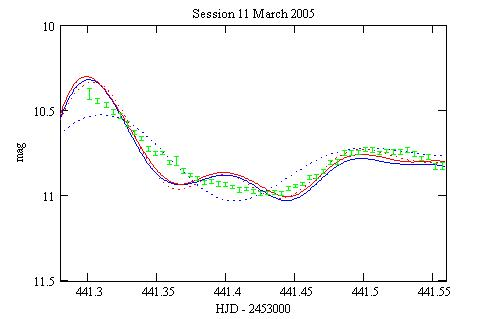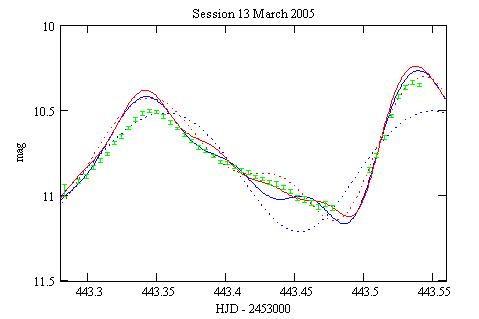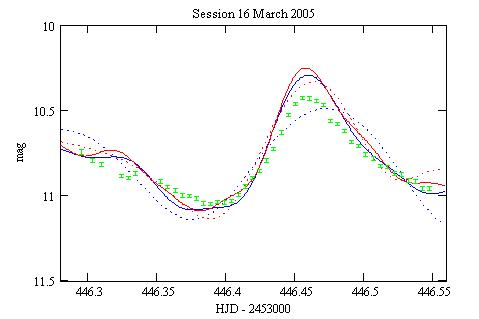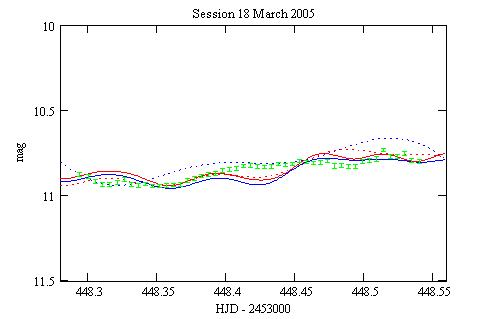With all 4 terms.

I have not tested the B magnitudes against the ephemeris, which is less detailled in this band.

### Conclusions

After some 50 yr the model of Fitch is still giving realistic results (with a 1.4 hour shift). This may be considered as remarkable considering the complicated behavior of VX Hya.

### Reference

Fitch W.S. (1966) ApJ 143 852.

### Technical notes

Telescope and camera configuration.

Computer and software configuration.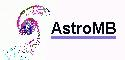Software for astronomy